Special Theory Of Relativity MCQ Level – 1

# Special Theory Of Relativity MCQ Level – 1

Test Description

## 10 Questions MCQ Test Topic wise Tests for IIT JAM Physics | Special Theory Of Relativity MCQ Level – 1

Special Theory Of Relativity MCQ Level – 1 for IIT JAM 2022 is part of Topic wise Tests for IIT JAM Physics preparation. The Special Theory Of Relativity MCQ Level – 1 questions and answers have been prepared according to the IIT JAM exam syllabus.The Special Theory Of Relativity MCQ Level – 1 MCQs are made for IIT JAM 2022 Exam. Find important definitions, questions, notes, meanings, examples, exercises, MCQs and online tests for Special Theory Of Relativity MCQ Level – 1 below.
Solutions of Special Theory Of Relativity MCQ Level – 1 questions in English are available as part of our Topic wise Tests for IIT JAM Physics for IIT JAM & Special Theory Of Relativity MCQ Level – 1 solutions in Hindi for Topic wise Tests for IIT JAM Physics course. Download more important topics, notes, lectures and mock test series for IIT JAM Exam by signing up for free. Attempt Special Theory Of Relativity MCQ Level – 1 | 10 questions in 30 minutes | Mock test for IIT JAM preparation | Free important questions MCQ to study Topic wise Tests for IIT JAM Physics for IIT JAM Exam | Download free PDF with solutions
 1 Crore+ students have signed up on EduRev. Have you?
Special Theory Of Relativity MCQ Level – 1 - Question 1

### A positive kaon (k+) has a rest mass of 494 MeV/c2 whereas a proton has a rest mass of 938 MeV/c2. If a koan has a total energy that is equal to the proton rest energy, speed of the kaon is most nearly :

Detailed Solution for Special Theory Of Relativity MCQ Level – 1 - Question 1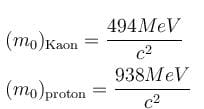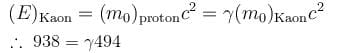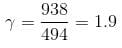On solving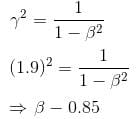The correct answer is: 0.85 c

Special Theory Of Relativity MCQ Level – 1 - Question 2

### A car of rest length 5 meters passes through a garage rest length 4 meters. Due to the relativistic Lorentz contraction, the car is only 3 meters long in the garage’s rest frame. There are doors on both ends of the garage, which open automatically when the front of the car reaches them and close automatically when the rear passes them. The opening or closing of each door requires a negligible amount of time. The velocity of the car in the garage’s rest frame is

Detailed Solution for Special Theory Of Relativity MCQ Level – 1 - Question 2

Length contraction: L0
with γ = 1/(1 − v2/c2)½

Given for the car, L0 = 5 m and = 3 m

γ = L0/L = 5/3 = 1/(1 − v2/c2)½
γ² = 25/9 = 1/(1 − v2/c2)
1 − v²/c² = 9/25
v²/c² = 1 − 9/25 = 16/25
4/c = 0.8c

Special Theory Of Relativity MCQ Level – 1 - Question 3

### The mean life of a meson is 2 x 10-8 sec. Calculate the mean life of a meson moving with a velocity 0.8c.

Detailed Solution for Special Theory Of Relativity MCQ Level – 1 - Question 3

∆t = t / √(1-v²/c²)

∆t = 2*10-8/√(1-(0.8)²)

∆t = 2*10-8 / 0.6

∆t = 3.3*10-8 s

Special Theory Of Relativity MCQ Level – 1 - Question 4

Two observers O and O' observe 2 events, A and B. The observers have a constant relative speed of 0.8c. In the units such that the speed of light is 1, observer O obtained the following coordinates.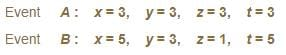What is the length of the space time interval between these 2 events, as measured by O' ?

Detailed Solution for Special Theory Of Relativity MCQ Level – 1 - Question 4

dS = dr+ (cdt) =  dxdydz− (cdt)
dS2 = dS'

dx2 = (5 − 3)2 = 4
dy2 = (3 − 3)2 = 0
dz2 = (1 − 3)2 = 4
dt2  = (5 − 3)2 = 4
c = 1

dS'= 4 + 0 + 4 − 4 = 4
dS' = 2

Special Theory Of Relativity MCQ Level – 1 - Question 5

The ultraviolet Lyman α line of hydrogen with wavelength 121.5 nm   is emitted by an astronomical object. An observer on earth measures the wavelength of the light received from the object to be 607.5 nm. The observer can conclude that the object is moving with a radial velocity of :

Detailed Solution for Special Theory Of Relativity MCQ Level – 1 - Question 5

Since the light received has a higher wavelength than the incident wavelength, one concludes that this is a red shift, that the object is moving away from the Earth.

where λ0 is the wavelength of the source.
The doppler effect equation gives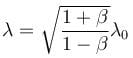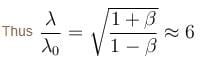Solving this one gets β = 35/37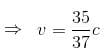v = 2.8 × 108 m/s
away from the Earth.

The correct answer is: 2.8 × 108 m/s away from Earth

Special Theory Of Relativity MCQ Level – 1 - Question 6

A particle leaving a cyclotron has a total relativistic energy of 10 GeV and a relativistic momentum of 8 GeV. What is the rest mass of this particle?

Detailed Solution for Special Theory Of Relativity MCQ Level – 1 - Question 6

Total energy = 10 GeV
p = 8 GeV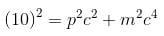In units in which c = 1 option (d)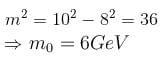The correct answer is: 6 GeV/c2

Special Theory Of Relativity MCQ Level – 1 - Question 7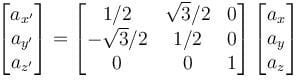The matrix shown above transforms the components of a vector in one coordinate frame S  to the components of the same vector in a second coordinate frame S'. This matrix represents a rotation of the reference frame S  by :

Detailed Solution for Special Theory Of Relativity MCQ Level – 1 - Question 7

The form of the matrix show that the rotation is about the z-axis

since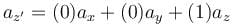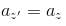since the z component does not change
∴  rotation about the z axis

Now, the standard from of the rotation matrix about z axis is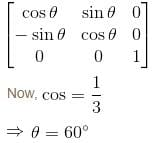The correct answer is: 60° counter clockwise about the z axis

Special Theory Of Relativity MCQ Level – 1 - Question 8

A car of rest length 5m  passes through a garage of rest length 4m. Due to the relativistic lorentz contraction, the car is only 3 long in length in the garage’s rest frame. There are doors on both ends of the garage, which open automatically when the front of the car reaches them and close automatically when the rear passes them. The opening or closing of the doors requires a negligible amount of time.

Which of the following is the best response to the question : “was the car ever inside the closed garage”

Detailed Solution for Special Theory Of Relativity MCQ Level – 1 - Question 8

Correct Answer :- d

Explanation : The order of the door opening depends on which reference frame one is in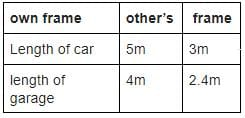So, the car fits in the garage’s frame (the car is 3m  and the garage is 4m). But in its own frame, the car is much longer to fit in the garage (car is 5m  and the garage is only 2.4m)

Both the frames give different answers.

There is no unique answer, as the order of door openings and closings depends on the reference frame.

Special Theory Of Relativity MCQ Level – 1 - Question 9

What is the length of a metre stick moving parallel to its length when its mass is 3/2 times of its rest mass.

Detailed Solution for Special Theory Of Relativity MCQ Level – 1 - Question 9

The mass of the rod is 3/2 times its rest mass, that is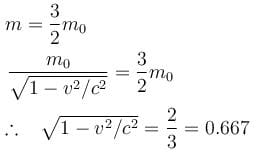The length of meter stick moving parallel to its length, according to length contraction formula.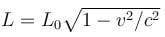L0 = 1m
L = 1 × 0.667 = 0.67m
The correct answer is: 0.67 m

Special Theory Of Relativity MCQ Level – 1 - Question 10

A space crew has a life support system that can last only for 1000 hours. What minimum speed would be required for safe travel of the crew between two space stations separated by a fixed distance of 1.08 x 1012 km ?

Detailed Solution for Special Theory Of Relativity MCQ Level – 1 - Question 10

1000 x 3600 x v / √ 1- v2/ c2 = 1.08 x 1012 x 1000

= 105 x 36 x v / √ 1- v2/ c2 = 1080 x 1012

= v / √ 1- v2/ c2 = c

v = c / √2

## Topic wise Tests for IIT JAM Physics

217 tests
 Use Code STAYHOME200 and get INR 200 additional OFF Use Coupon Code
Information about Special Theory Of Relativity MCQ Level – 1 Page
In this test you can find the Exam questions for Special Theory Of Relativity MCQ Level – 1 solved & explained in the simplest way possible. Besides giving Questions and answers for Special Theory Of Relativity MCQ Level – 1, EduRev gives you an ample number of Online tests for practice

217 tests